﻿ Objects > Iterations and Iterated Images

# Iterations and Iterated ImagesAn iteration is a rule that defines a repeated series of geometric or numeric operations. Each step of the iteration is performed on the results of the previous step, and the iteration rule defines how the results of one step are used to perform the next step.

An iterated image object is the set of iterated objects corresponding to a single constructed or calculated object from the original operation.

Use the command to create an iteration.

An iteration can involve geometric constructions, numeric operations, or a combination of both.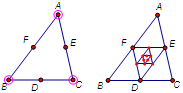Geometric Iteration: Midpoint Triangles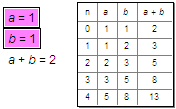Numeric Iteration: The Fibonacci Sequence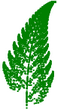Combined Iteration: A Fractal Fern

The geometric constructions or numeric operations must define an output in terms of some input. The input can be independent points, points on a path, parameters or independent calculations. The iteration uses the output of one step as the input for the next step, and creates an iterated image object for each output object. The iterated image object shows the set of iterated positions or values of the original object on which it’s based. This is called the orbit of that object.

 A geometric iteration repeatedly applies a particular geometric construction to a set of points. At each step the construction is based on the point(s) produced by the previous step. For instance, you could construct a triangle from three points and then translate the original points to the right by 2 cm. When you iterate this construction, the first step constructs a new triangle from the translated points and produces three new translated points. Each additional iteration produces a new triangle and three new translated points. The sequence of triangles is the orbit of the original triangle you constructed.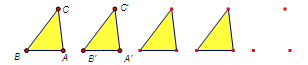A numeric iteration repeatedly applies a particular calculation. At each step the calculation uses the result(s) of the previous step. For instance, you can apply the calculation “add 2” repeatedly, starting with the seed value a = 5. The first step produces 5 + 2 = 7, the second step produces 7 + 2 = 9, and so forth. The sequence of output values (7, 9, 11, 13, ...) is the orbit of a + 2. Sketchpad displays the orbit of iterated numeric or algebraic objects in a table. The first column shows the depth of iteration; additional columns show the orbits of the iterated numeric or algebraic objects.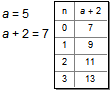In these examples, it’s helpful to think of the iteration as having two distinct elements: the original calculation or construction (the seed or pre-image) and the mapping that takes each value or point to the next value or point in the sequence. Thus you might say that 5 maps to 7 in the numeric iteration, and that 47 maps to 49. Similarly, you might say that A maps to A' in the geometric iteration, and that A' maps to the next translated point. The entire iteration is defined by the pre-image (the seed) and the mapping. When you first apply the mapping to the pre-image, the result is the first image. As you continue to apply the mapping, you generate the second image, the third image, thes fourth image, and so on.

 Most iterations use a single mapping to produce a single image of the original for each step of the iteration. However, some iterations (like this Sierpiński Gasket) produce multiple copies at each step.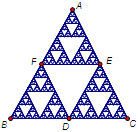When you construct an iteration, Sketchpad creates iterated image objects of the objects produced by the original construction, even those objects that aren’t used as input for the next step of the iteration. For instance, in the geometric example above, Sketchpad creates iterated image objects not only of the three vertices of the triangle, but also of the segments and triangle interior. Objects that can be iterated include geometric objects, measurements, loci, functions and function plots, pictures, and text attached to a point. (Sketchpad does not produce iterated image objects for families of functions, for families of loci, for action buttons, or for other previously-constructed iterated images.)